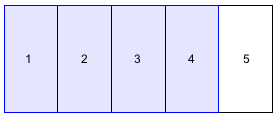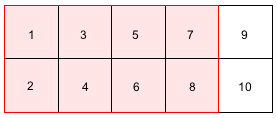### Home > AC > Chapter 1 > Lesson 1.1.3 > Problem1-25

1-25.

In Algebra, you will need to be able to work with numbers, words, and geometric representations. Use these representations to answer the following questions.

1. Write another fraction that is equivalent to $\frac{4}{5}$. Draw diagrams to show that they are equal.

Try multiplying by a fraction equal to $1$.$\frac{4}{5}\cdot \frac{2}{2}=\frac{8}{10}$

2. Find the equivalent decimal for both fractions. Was rounding your answer necessary?

$\frac{8}{10}=0.8$

Rounding was not necessary.

3. Find the equivalent percent for both fractions.

Percent means 'per hundred' or 'out of a hundred'.

Try setting it up as a proportion to $100$.

$\frac{4}{5}=\frac{x}{100}$

Multiply the top and bottom by $20$ to make the denominator equal to $100$ and solve for $x$.

$\frac{4}{5}\cdot \frac{20}{20}=\frac{80}{100}$

$x=80$ so, $x=80\%$